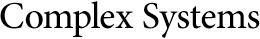## Period-Halving Bifurcation of a Neuronal Recurrence Equation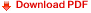René Ndoundam
University of Yaounde I, UMI 209, UMMISCO
P.O. Box 337 Yaounde, Cameroon
and
University of Yaounde I, LIRIMA
Team GRIMCAPE, Faculty of Science, Department of Computer Science
P.O. Box 812 Yaounde, Cameroonndoundam@gmail.com

#### Abstract

The sequences generated by neuronal recurrence equations of the form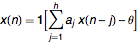are studied. From a neuronal recurrence equation of memory size h that describes a cycle of length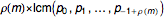, a set of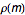neuronal recurrence equations is constructed with dynamics that describe respectively the transient of length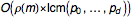and the cycle of length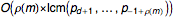if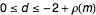and 1 if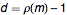. This result shows the exponential time of the convergence of the neuronal recurrence equation to fixed points and the existence of the period-halving bifurcation.

https://doi.org/10.25088/ComplexSystems.20.4.325